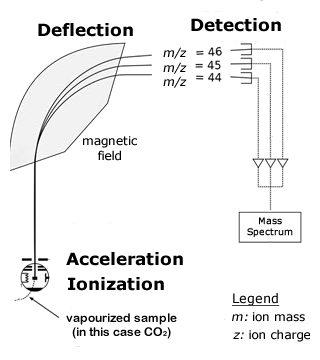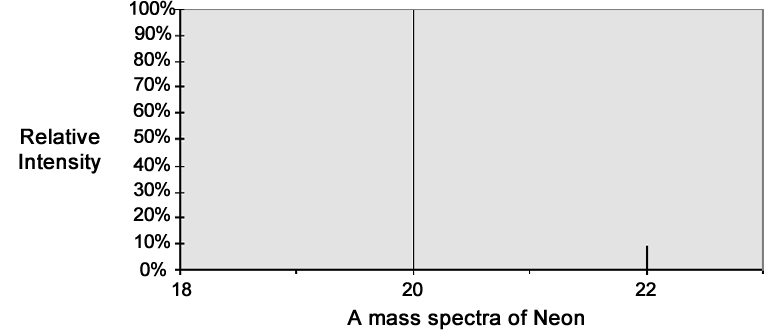About the Instrument
A mass spectrometer is an instrument that is capable of measuring the mass - to - charge ratio of individual molecules that have been electrically charged to form ions. Often, the ions have a charge of only 1 (z = 1), so the mass-to-charge ratio is actually the same as the mass of the ion.

In a mass spectrometer, ions are accelerated to a high speed in an electric field and then shot into a magnetic field, which applies a force perpendicular to the ions\' direction of travel. The force from the magnetic field deflects ions to different degrees, depending on their mass-to-charge ratio. Lighter ions are deflected more than heavier ones, so when they reach the detector at the other end of the mass spectrometer, it measures the deflection of each ion beam. This measurement can be used to calculate the mass-to-charge ratio of the ions, which allows the chemical and isotopic composition of the sample to be determined.

Isotope Ratio Mass Spectrometry is used to find the isotopic composition of elements within a sample. These instruments are very sensitive, because they have to measure very small differences in masses and the abundance of very rare isotopes. They usually have a single magnet that bends a beam of ions towards a group of Faraday cups, which capture the ions and convert their energy to electric current.Working with the Units
The numbers outputted from a mass spectrometer are usually values of % relative abundance or intensity. For example, in the mass spectra of neon (right), there are three different ions: mass 20, with a relative abundance of 100%; 21, with a relative abundance of 0.3% (so small that it does not even show up on the graph); and 22, with relative abundances of 8.82%.

Since isotopic ratios are generally very small, it is often easier to convert them into delta (or del) notation. This is calculated based on an international standard for the particular element you are studying, and is expressed in units of permil (‰, similar to percent, except that it is per thousand units instead of per hundred). This conversion requires the following equation.
δ = (Rsample - Rstandard / Rstandard) • 1000
Rsample is the isotopic ratio of your sample, and Rstandard is a standard ratio based on the internationally accepted reference (for example, the Rstandard value of 13C/12C is based on the fossil Pee Dee Belemnite).